Test: Single Phase HW AC-DC - 1

# Test: Single Phase HW AC-DC - 1 - Electrical Engineering (EE)

Test Description

## 20 Questions MCQ Test Power Electronics - Test: Single Phase HW AC-DC - 1

Test: Single Phase HW AC-DC - 1 for Electrical Engineering (EE) 2023 is part of Power Electronics preparation. The Test: Single Phase HW AC-DC - 1 questions and answers have been prepared according to the Electrical Engineering (EE) exam syllabus.The Test: Single Phase HW AC-DC - 1 MCQs are made for Electrical Engineering (EE) 2023 Exam. Find important definitions, questions, notes, meanings, examples, exercises, MCQs and online tests for Test: Single Phase HW AC-DC - 1 below.
Solutions of Test: Single Phase HW AC-DC - 1 questions in English are available as part of our Power Electronics for Electrical Engineering (EE) & Test: Single Phase HW AC-DC - 1 solutions in Hindi for Power Electronics course. Download more important topics, notes, lectures and mock test series for Electrical Engineering (EE) Exam by signing up for free. Attempt Test: Single Phase HW AC-DC - 1 | 20 questions in 20 minutes | Mock test for Electrical Engineering (EE) preparation | Free important questions MCQ to study Power Electronics for Electrical Engineering (EE) Exam | Download free PDF with solutions
 1 Crore+ students have signed up on EduRev. Have you?
Test: Single Phase HW AC-DC - 1 - Question 1

### A voltage source Vs = Vm sinωt is connected in series with a resistance R and an SCR. At some firing angle delay of α a positive gate pulse is applied to the SCR which turns on the SCR. Considering ideal conditions, at the instant α the voltage at the resistor terminals Vo

Detailed Solution for Test: Single Phase HW AC-DC - 1 - Question 1

At the firing angle, the SCR turns on and thus the resistor or load R is connected to the source.

Test: Single Phase HW AC-DC - 1 - Question 2

### The average output voltage is maximum when SCR is triggered at ωt =

Detailed Solution for Test: Single Phase HW AC-DC - 1 - Question 2

The sooner the conduction starts higher the average power. Though practically a device cannot be triggered at exactly zero degrees.

Test: Single Phase HW AC-DC - 1 - Question 3

### In the below given circuit, the SCR is triggered at a certain firing angle α.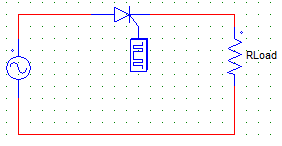The load current falls to zero at ωt =

Detailed Solution for Test: Single Phase HW AC-DC - 1 - Question 3

The SCR is naturally commutated at 90°.

Test: Single Phase HW AC-DC - 1 - Question 4

In the method of phase control, the phase relationship between ___ & ___ is controlled by varying the firing angle

Detailed Solution for Test: Single Phase HW AC-DC - 1 - Question 4

By varying the firing angle, the load current and load voltage can be controlled.

Test: Single Phase HW AC-DC - 1 - Question 5

In a single phase half-wave thyristor circuit with R load & Vs=Vm sinωt, the maximum value of the load current can be given by

Detailed Solution for Test: Single Phase HW AC-DC - 1 - Question 5

Vm is the peak value of the load as well as supply voltage. I = Vm/R.

Test: Single Phase HW AC-DC - 1 - Question 6

Below shown ideal circuit is triggered at a firing angle of α. The voltage across the SCR is zero from ωt =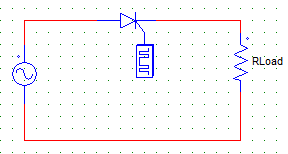Detailed Solution for Test: Single Phase HW AC-DC - 1 - Question 6

Before the SCR is triggered all of the supply voltage appears across it that is from 0 to α, at α the device is gated and it starts to conduct acting like a S.C (ideally). Hence voltage across it is zero from α to π. At π the SCR is again naturally commutated due to the reversal of the AC supply & a negative voltage appears across it.

Test: Single Phase HW AC-DC - 1 - Question 7

Below shown ideal circuit is triggered at a firing angle of α. From ωt = π to 2π+α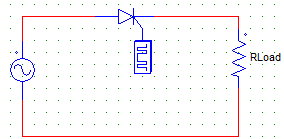Detailed Solution for Test: Single Phase HW AC-DC - 1 - Question 7

From ωt = π to 2π+α, negative cycle is active and the SCR experiences revered biased voltage by Vs.

Test: Single Phase HW AC-DC - 1 - Question 8

For a single phase thyristor circuit with R load & firing angle α, the conduction angle can be given by

Detailed Solution for Test: Single Phase HW AC-DC - 1 - Question 8

SCR is fired at α, hence it conducts from α till it is commutated by the reversal of the AC supply i.e at π radians.

Test: Single Phase HW AC-DC - 1 - Question 9

For the below given circuit with firing angle α & angular frequency of ω, the circuit turn-off time in secs can be given by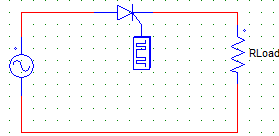Detailed Solution for Test: Single Phase HW AC-DC - 1 - Question 9

The circuit turns of at ωt = π as it is a half wave circuit.
Hence toff = π/ω.

Test: Single Phase HW AC-DC - 1 - Question 10

A single-phase half-wave thyristor circuit with R load is triggered at an angle of α = 0°. As such, the maximum value of the average output voltage would be given byConsider Vs = Vm sinωt.

Detailed Solution for Test: Single Phase HW AC-DC - 1 - Question 10

For the whole cycle of 2π, the R load would be active only for half of the cycle. Hence, Vo = Vm/π.

Test: Single Phase HW AC-DC - 1 - Question 11

For a certain SCR configuration, the below shown waveform is obtained. Find the value of the average output voltage with α = 30° & Vs = 240 V.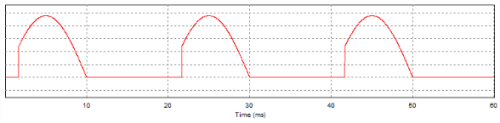Detailed Solution for Test: Single Phase HW AC-DC - 1 - Question 11

Vm = √2 Vs = 339.41 V
Vavg = ∫ Vm Sin ωt d(ωt) where the integration runs from α to π
Vavg = Vm/2π (1+cos α).

Test: Single Phase HW AC-DC - 1 - Question 12

A single-phase half wave circuit has Vs = 230 V with a R load of 100 Ω. Find the average load current at α = 30°.

Detailed Solution for Test: Single Phase HW AC-DC - 1 - Question 12

Io = Vo/R
Vo = (Vm/2π) x (1+cosα).

Test: Single Phase HW AC-DC - 1 - Question 13

A single phase 230 V, 1 kW heater is connected across a 1-phase 230 V, 50 Hz supply through an SCR. The firing angle of the SCR is adjusted to give a rms voltage of 155 V. Find the power absorbed by the heater element.

Detailed Solution for Test: Single Phase HW AC-DC - 1 - Question 13

R (Heater element resistance) = 2302/1kW = 52.9 Ω
Now, P = Vrms2/R = 1552/52.9.

Test: Single Phase HW AC-DC - 1 - Question 14

For a single phase half-wave thyristor circuit with R load, the power delivered to the resistive load is

Detailed Solution for Test: Single Phase HW AC-DC - 1 - Question 14

P = I2.R
V = IR
I = V/R
Hence, P = V2/R.

Test: Single Phase HW AC-DC - 1 - Question 15

For a single phase half-wave thyristor circuit with R load, the input power factor is given by

Detailed Solution for Test: Single Phase HW AC-DC - 1 - Question 15

(p.f)input = power delivered to load/input VA.

Test: Single Phase HW AC-DC - 1 - Question 16

For the below shown circuit,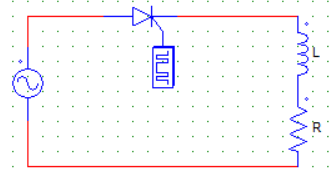The load voltage waveform from ωt = π to ωt = π+α = β (extinction angle) is

Detailed Solution for Test: Single Phase HW AC-DC - 1 - Question 16

In this case, the Inductor L is force conducting the SCR & supply current to the source the current is gradually decaying as the inductor is discharging.

Test: Single Phase HW AC-DC - 1 - Question 17

For the below shown circuit,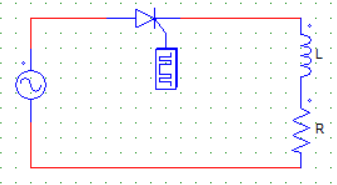The SCR is gated at α. At ωt = π

Detailed Solution for Test: Single Phase HW AC-DC - 1 - Question 17

At π the L force conducts the SCR & current starts gradually decaying whereas at π the voltage is zero as it goes from positive to negative at π.

Test: Single Phase HW AC-DC - 1 - Question 18

In case of a single-phase half-wave circuit with RL load, with firing angle α and extinction angle β, the conduction angle γ can be written as

Detailed Solution for Test: Single Phase HW AC-DC - 1 - Question 18

Extinction angle is some angle > π at which load current reduces to zero. It depends upon the inductor value.

Test: Single Phase HW AC-DC - 1 - Question 19

For a half-wave single phase thyristor rectifier circuit with any type of load the _______ + _________ waves must ideally give the supply voltage waveform.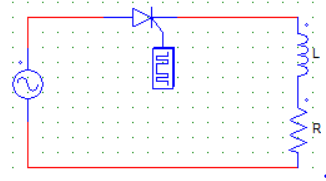Detailed Solution for Test: Single Phase HW AC-DC - 1 - Question 19

The supply voltage either appears across at the thyristor or the load.

Test: Single Phase HW AC-DC - 1 - Question 20

In case of a single-phase half-wave circuit with RL load, with firing angle α and extinction angle β, the thyristor is reversed biased from

Detailed Solution for Test: Single Phase HW AC-DC - 1 - Question 20

As the load current goes to zero from β, the SCR is reversed biased or in the OFF state till it is turned on at 2π again by the positive AC cycle.

## Power Electronics

5 videos|39 docs|63 tests
Information about Test: Single Phase HW AC-DC - 1 Page
In this test you can find the Exam questions for Test: Single Phase HW AC-DC - 1 solved & explained in the simplest way possible. Besides giving Questions and answers for Test: Single Phase HW AC-DC - 1, EduRev gives you an ample number of Online tests for practice

## Power Electronics

5 videos|39 docs|63 tests(Scan QR code)